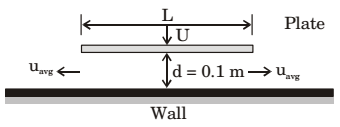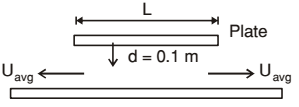## Fluid Mechanics and Hydraulic Machinery Miscellaneous

#### Fluid Mechanics and Hydraulic Machinery

1.  Water flows though a pipe with a velocity given by V→ =4 + x + yĵ m/s t

m/s, where ĵ is the unit vector in the y direction, t(>0) is in seconds, and x and y are in meters. The magnitude of total acceleration at the point (x, y) = (1, 1) at t = 2 s is _____ m/s².

1. Given:

 V =4 + x + yĵ m/s t

V = uî + vĵ + wk̂
u = 0, w = 0
 V =4 + x + yĵ t

a = axî + ayĵ + az
 ax = u ∂u + v ∂u + w ∂u + ∂u = 0 ∂x ∂y ∂z ∂t

 az = u ∂w + v ∂w + w ∂w + ∂w ∂x ∂y ∂z ∂t

 ay = u ∂v + v ∂v + w ∂v + ∂v ∂x ∂y ∂z ∂t

 = u ∂v + v ∂v ∂y ∂t

 =4 + x + y× 1 +4t t²

 ay =4 + x + y − 4ĵ t t²

Now, At (x,y) = 1,1 and t = 2 sec.
Total acceleration is given by,
 a = ay =4 + 1 + 1 − 4= 3 m/s² 2 4

##### Correct Option: A

Given:

 V =4 + x + yĵ m/s t

V = uî + vĵ + wk̂
u = 0, w = 0
 V =4 + x + yĵ t

a = axî + ayĵ + az
 ax = u ∂u + v ∂u + w ∂u + ∂u = 0 ∂x ∂y ∂z ∂t

 az = u ∂w + v ∂w + w ∂w + ∂w ∂x ∂y ∂z ∂t

 ay = u ∂v + v ∂v + w ∂v + ∂v ∂x ∂y ∂z ∂t

 = u ∂v + v ∂v ∂y ∂t

 =4 + x + y× 1 +4t t²

 ay =4 + x + y − 4ĵ t t²

Now, At (x,y) = 1,1 and t = 2 sec.
Total acceleration is given by,
 a = ay =4 + 1 + 1 − 4= 3 m/s² 2 4

1. Consider the two-dimensional velocity field given by V = (5 + a1x + +b1y) ˆ i + (4 + a2x + b2y) ˆ j, where a1, b1, a2 and b2 are constants. Which one of the following conditions needs to be satisfied for the flow to be incompressible?

1. For continuous and incompressible flow
ux + uy = 0
a1 + b2 = 0

##### Correct Option: B

For continuous and incompressible flow
ux + uy = 0
a1 + b2 = 0

1. A flow field which has only convective acceleration is

1. Convective acceleration is the effect of time independent acceleration of fluid with respect to space that means flow is steady non-uniform flow.

##### Correct Option: C

Convective acceleration is the effect of time independent acceleration of fluid with respect to space that means flow is steady non-uniform flow.

1. In a two-dimensional velocity field with velocities u and v along the x and y directions respectively, the convective acceleration along the x-direction is given by

1. Two dimensional velocity field with velocities u, v and along x and y direction.
∴  Acceleration along x direction, ax = aconvective + atemporal or local

 = u ∂u + v ∂u + w ∂u + ∂u ∂x ∂y ∂z ∂t

Since, (∂u/∂z) = 0 for 2-dimensional field, therefore
 Convective acceleration u ∂u + v ∂u ∂x ∂y

##### Correct Option: C

Two dimensional velocity field with velocities u, v and along x and y direction.
∴  Acceleration along x direction, ax = aconvective + atemporal or local

 = u ∂u + v ∂u + w ∂u + ∂u ∂x ∂y ∂z ∂t

Since, (∂u/∂z) = 0 for 2-dimensional field, therefore
 Convective acceleration u ∂u + v ∂u ∂x ∂y

1. A flat plate of width L = 1 m is pushed down with a velocity U = 0.01 m/s towards a wall resulting in the drainage of the fluid between the plate and the wall as shown in the figure. Assume two- dimensional incompressible flow and that the plate remains parallel to the wall. The average velocity, uavg of the fluid (in m/s) draining out at the instant shown in the figure is _____ (correct to three decimal places).1.By mass conservation:- 2(s × uavg × db) = (s. u × Lb)
⇒  2 × vavg × 0.1 = 0.01 × 1
uavg = 0.05 m/s

##### Correct Option: CBy mass conservation:- 2(s × uavg × db) = (s. u × Lb)
⇒  2 × vavg × 0.1 = 0.01 × 1
uavg = 0.05 m/s